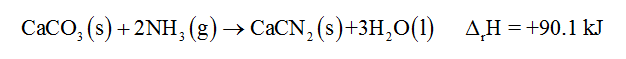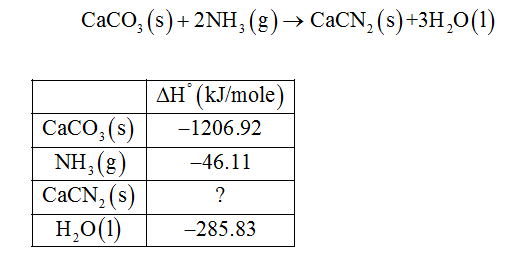# When one mole of calcium carbonate reacts with ammonia, solid calcium cyanamide, CaCN2, and liquid water are formed. The reaction absorbs 90.1 kJ of heat. Write a balanced thermochemical equation for the reaction. Calculate ΔHf° for calcium cyanamide

Question
16 views

When one mole of calcium carbonate reacts with ammonia, solid calcium cyanamide, CaCN2, and liquid water are formed. The reaction absorbs 90.1 kJ of heat. Write a balanced thermochemical equation for the reaction. Calculate ΔHf° for calcium cyanamide

check_circle

Step 1

Given that calcium carbonate reacts with ammonia to form solid calcium cyanamide and also given that reaction absorbs 90.1 k J of heat. The balanced equation for the reaction is,Step 2

Given the reaction and standard enthalpy of formation of reactant and reaction and using these values, enthalpy of formation for the given product can be determined....

### Want to see the full answer?

See Solution

#### Want to see this answer and more?

Solutions are written by subject experts who are available 24/7. Questions are typically answered within 1 hour.*

See Solution
*Response times may vary by subject and question.
Tagged in